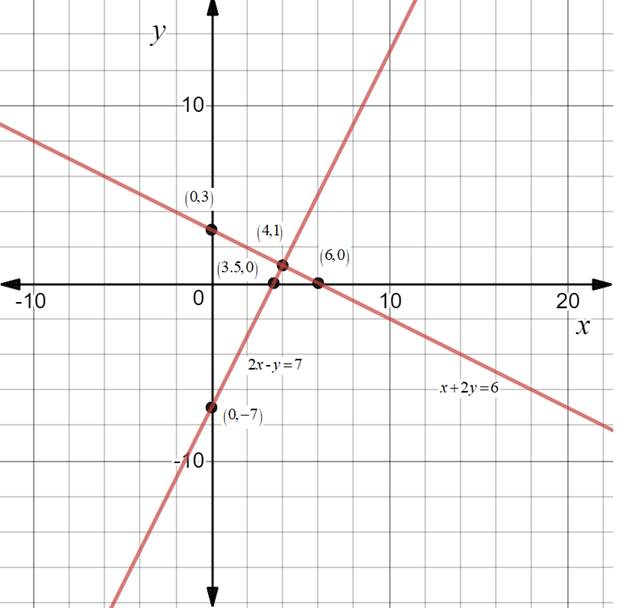Chapter 10.CT, Problem 18CTElementary Geometry For College St...

7th Edition
Alexander + 2 others
ISBN: 9781337614085

Solutions

Chapter
SectionElementary Geometry For College St...

7th Edition
Alexander + 2 others
ISBN: 9781337614085
Textbook Problem

Use the graphs provided to solve the system consisting of the equations x + 2 y = 6 and 2 x - y = 7 .To determine

To solve:

The system consisting of the equations x+2y=6 and 2x-y=7 using the given graph.Explanation

It is observed from the graph that the point of intersection is (4,1)

Still sussing out bartleby?

Check out a sample textbook solution.

See a sample solution

The Solution to Your Study Problems

Bartleby provides explanations to thousands of textbook problems written by our experts, many with advanced degrees!

Get Started

Find more solutions based on key concepts